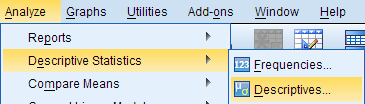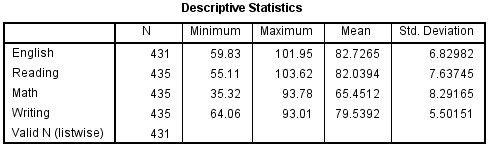# SPSS Tutorials: Descriptive Stats for Many Numeric Variables (Descriptives)

Descriptives is best to obtain quick summaries of numeric variables, or to compare several numeric variables side-by-side.

## Introduction

When summarizing quantitative (continuous/interval/ratio) variables, we are typically interested in questions like:

• What is the "center" of the data? (Mean, median)
• How spread out is the data? (Standard deviation/variance)
• What are the extremes of the data? (Minimum, maximum; Outliers)
• What is the "shape" of the distribution? Is it symmetric or asymmetric? Are the values mostly clustered about the mean, or are there many values in the "tails" of the distribution? (Skewness, kurtosis)

In SPSS, the Descriptives procedure computes a select set of basic descriptive statistics for one or more continuous numeric variables. In all, the statistics it can produce are:

• N valid responses
• Mean
• Sum
• Standard deviation
• Variance
• Minimum
• Maximum
• Range
• Standard error of the mean (or S.E. mean)
• Skewness
• Kurtosis

Additionally, the Descriptives procedure can optionally compute Z scores as new variables in your dataset.

Notice that the Descriptives procedure can't compute medians or quartiles. If you need those statistics, you'll need to use the Frequencies, Explore, or Compare Means procedures. The Descriptives procedure is best used when you want to compare the descriptive statistics of several numeric variables side-by-side.

## Descriptives

To run the Descriptives procedure, select Analyze > Descriptive Statistics > Descriptives.The Descriptives window lists all of the variables in your dataset in the left column. To select variables for analysis, click on the variable name to highlight it, then click on the arrow button to move the variable to the column on the right. Alternatively, you can double-click on the name of a variable to move it to the column on the right.Selecting the Save standardized values as variables check box will compute new variables containing the standardized values (also known as Z scores) of each of the input variables. Recall that the standardized value of a variable is computed by subtracting its mean and then dividing that difference by the standard deviation:

$$Z = \frac{X - \mu}{\sigma}$$

By default, the Descriptives procedure computes the mean, standard deviation, minimum, and maximum of the variable. Clicking Options will allow you to disable any of the aforementioned statistics, or enable sum, variance, range, standard error of the mean (S.E. mean), kurtosis, and skewness. You can also choose how you want the output to be organized:

• Variable list will print the variables in the same order that they are specified in the Descriptives window.
• Alphabetically will arrange the variables in alphabetical order.
• Ascending means will order the output so that the variables with the smallest means are first and the variables with the largest means last.
• Descending means will order the output so that the variables with the largest means are first and the variables with the smallest means are last.The Descriptives dialog window will let you enter any numeric variables in your dataset -- including nominal and ordinal variables. Means and standard deviations are not appropriate or meaningful for nominal and ordinal variables. Make sure that you understand how your variables are coded and what they represent before you start your analysis.

## Example: Comparing average test scores side-by-side

### Problem Statement

The sample dataset has test scores (out of 100) on four placement tests: English, Reading, Math, and Writing. We want to compare the summary statistics of these four tests so we can determine which tests the students tended to do the best and the worst on.

### Running the Procedure

#### Using the Descriptives Dialog Window

1. Click Analyze > Descriptive Statistics > Descriptives.
2. Double click on the variables English, Reading, Math, and Writing in the left column to move them to the Variables box.
3. Click OK when finished.

#### Using Syntax

DESCRIPTIVES VARIABLES=English Reading Math Writing
/STATISTICS=MEAN STDDEV MIN MAX.

### OutputHere we see a side-by-side comparison of the descriptive statistics for the four numeric variables. This allows us to quickly make the following observations about the data:

• Some students were missing scores for the English test.
• The maximum scores observed on the English and the Reading tests exceed 100 points, which was supposed to be the maximum possible score. This could indicate a problem with data entry, or could indicate an issue with the scoring method. Before proceeding with any other data analysis, we would need to resolve the issues with these measurements.
• The minimum Math score was far lower than the minimum scores for the other sections of the test.
• The averages of the English and Reading scores were very close.
• Math had the lowest average score of the four sections, but the highest standard deviation in scores.

## Example: Computing and Interpreting Z-Scores

Recall that a Z score for an observation of some variable X is computed as

$$Z_i = \frac{X_i - \mu}{\sigma}$$

where

xi is the ith observed value of X
μ is the population mean of X
σ is the population standard deviation of X
Zi is the computed z-score corresponding to xi.

Stated another way, a Z score is simply an observation that has been centered about its mean and scaled to its standard deviation. The end result is a standardized version of the variable, whose units are now in terms of "standard deviation units". (A Z score of 1 means that it is one standard deviation above the mean; a Z score of -1 means that it is one standard deviation below the mean.)

In SPSS, you can compute standardized scores for numeric variables automatically using the Descriptives procedure. One important distinction is that the standardized values of the "raw" scores will be centered about their sample means and scaled (divided by) their sample standard deviations; that is:

$$z = \frac{x - \bar{x}}{s}$$

### Running the Procedure

#### Using the Descriptives Dialog Window

1. Click Analyze > Descriptive Statistics > Descriptives.
2. Add the variables English, Reading, Math, and Writing to the Variables box.
3. Check the box Save standardized values as variables.
4. Click OK when finished.

#### Using Syntax

DESCRIPTIVES VARIABLES=English Reading Math Writing
/SAVE
/STATISTICS=MEAN STDDEV MIN MAX.

### Output

If the computation is successful, then the syntax will be printed in the Output window, and new variables called ZEnglish, ZReading, ZMath, and ZWriting will be added to the Data View.

If you want to compute Z scores for a variable using a known population mean and population standard deviation, use the Compute Variables procedure instead, and enter the Z score formula using the desired population mean and standard deviation values in the expression.